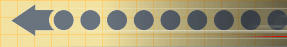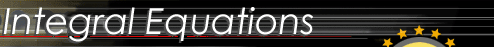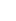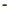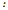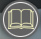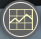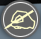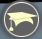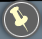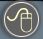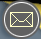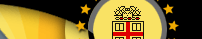Mathematical techniques involving differential equations used in the analysis of physical, biological and economic phenomena. Emphasis is placed on the use of established methods, rather than rigorous foundations.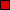First and second order differential equations.Series solutions of second order linear equations.The Laplace transform.Numerical methods.    This course in elementary differential equations is designed to introduce the student for displaying the interrelations between mathematics and physical sciences or engineering. The principal attention is given to those methods that are capable of broad applications and that can be extended to various problems.    The methods discussed here include not only elementary analytical techniques that lead to exact solutions of certain classes of problems, but also include approximations based on numerical algorithms or series expansions, as well as qualitative or geometrical methods. The problem sets will be assigned and discussed in subsequent class sessions. A student is expected to learn how to use a software package (such as Maple, Matlab, Mathematica, etc.) in solving ordinary differential equations. Prerequisite: Elementary Calculus.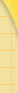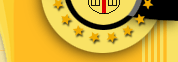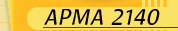ReadingSyllabusGradingExams & SolutionsHomeworkUtilities/softwareapplicationsContactComputingMain WebsiteAPMA0330APMA0340APMA1210APMA2140